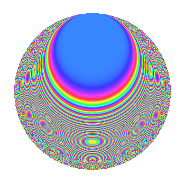# Properties

 Label 210.3.kLevel 210 Weight 3 Character orbit k Rep. character $$\chi_{210}(83,\cdot)$$ Character field $$\Q(\zeta_{4})$$ Dimension 64 Newform subspaces 2 Sturm bound 144 Trace bound 2

# Related objects

## Defining parameters

 Level: $$N$$ $$=$$ $$210 = 2 \cdot 3 \cdot 5 \cdot 7$$ Weight: $$k$$ $$=$$ $$3$$ Character orbit: $$[\chi]$$ $$=$$ 210.k (of order $$4$$ and degree $$2$$) Character conductor: $$\operatorname{cond}(\chi)$$ $$=$$ $$105$$ Character field: $$\Q(i)$$ Newform subspaces: $$2$$ Sturm bound: $$144$$ Trace bound: $$2$$ Distinguishing $$T_p$$: $$23$$

## Dimensions

The following table gives the dimensions of various subspaces of $$M_{3}(210, [\chi])$$.

Total New Old
Modular forms 208 64 144
Cusp forms 176 64 112
Eisenstein series 32 0 32

## Trace form

 $$64q - 8q^{7} + O(q^{10})$$ $$64q - 8q^{7} - 24q^{15} - 256q^{16} + 32q^{18} + 24q^{21} - 80q^{22} + 32q^{25} - 16q^{28} + 96q^{30} - 80q^{36} + 64q^{37} - 64q^{42} - 64q^{43} - 96q^{46} + 168q^{51} + 584q^{57} + 224q^{58} - 32q^{60} + 240q^{63} + 64q^{67} - 64q^{72} - 128q^{78} + 152q^{81} - 80q^{85} - 160q^{88} - 544q^{91} - 840q^{93} + O(q^{100})$$

## Decomposition of $$S_{3}^{\mathrm{new}}(210, [\chi])$$ into newform subspaces

Label Dim. $$A$$ Field CM Traces $q$-expansion
$$a_2$$ $$a_3$$ $$a_5$$ $$a_7$$
210.3.k.a $$32$$ $$5.722$$ None $$-32$$ $$0$$ $$0$$ $$-4$$
210.3.k.b $$32$$ $$5.722$$ None $$32$$ $$0$$ $$0$$ $$-4$$

## Decomposition of $$S_{3}^{\mathrm{old}}(210, [\chi])$$ into lower level spaces

$$S_{3}^{\mathrm{old}}(210, [\chi]) \cong$$ $$S_{3}^{\mathrm{new}}(105, [\chi])$$$$^{\oplus 2}$$

## Hecke characteristic polynomials

There are no characteristic polynomials of Hecke operators in the database